Refer to our Texas Go Math Grade 4 Answer Key Pdf to score good marks in the exams. Test yourself by practicing the problems from Texas Go Math Grade 4 Lesson 9.1 Answer Key Remainders.

Essential Question

How can you use arrays to divide whole numbers that do not divide evenly?
It is easy to apply operation on different numbers of elements of an array.
First, we provide values like the number of elements and array.
Then a number
to divide all elements of an array using a for a loop.
Then we are going
to display the final numbers.
When a number cannot be divided evenly, the amount left over is called the remainder.
Explanation:Investigate

Materials: square tiles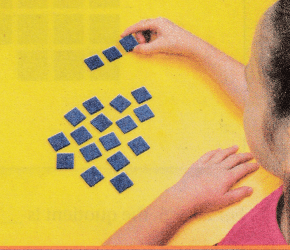Erica and 2 friends are playing a game of dominoes. There are 19 dominoes in the set. Erica wants each player to receive the same number of dominoes. Can she divide them equally among the 3 players? Why or why not?
No, she divide them equally among the 3 players.
Explanation:
19 dominoes can not be divided in to 3 equal parts,
when we divide 1 is left over.

You can use an array to find the number of dominoes each player will receive.

A. Use 19 tiles to represent the 19 dominoes. Make an array to find how many rows of 3 are in 19 and if any are left over.
19 ÷ 3
3 x 6 + 1
18 + 1
Explanation: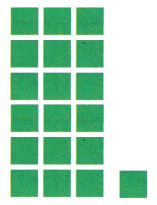6 rows and 1 is left over.

B. Make a row of 3 tiles.
6 rows and 1 left overExplanation:
19 ÷ 3
3 x 6 + 1
18 + 1

C. Continue to make as many rows of 3 tiles as you can.Explanation:
19 ÷ 3
3 x 6 + 1
18 + 1 = 19

D. Find the number of rows of three tiles and the number of tiles left over. Record your answer.
__________ rows of 3 tiles
__________ tile left over
So, each player gets __________ dominoes with __________ domino left over.
6 rows of 3 tiles
1 tile left over
So, each player gets 6 dominoes with 1 domino left over.
Explanation:
Total dominoes are 19
6 rows of 3 tiles 18 tiles
1 tile left over
So, each player gets 6 dominoes with 1 domino left over.

Math Talk

Mathematical Processes
Explain how the model helped you find the number of dominoes each player receives Why is 1 tile left over.
The mode is easy to apply operation on different numbers of elements of an array.
First, we provide values like the number of elements and array.
Then a number
to divide all elements of an array using a for a loop.
Then we are going
to display the final numbers.

Make Connections

When a number cannot be divided evenly, the amount left over is called the remainder.

Use square tiles to find 29 ÷ 5.

• Use 29 tiles.
• Use the tiles to make rows of 5. The number of tiles left the remainder.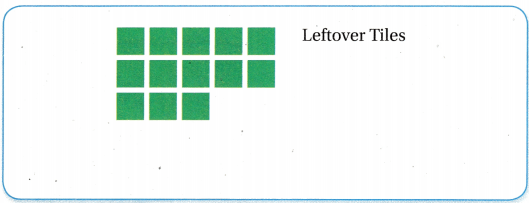For 29 ÷ 5, the quotient is __________ and the remainder is __________, or 5 r 4.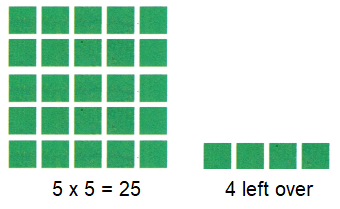Explanation:
29 ÷ 5, the quotient is 5 and the remainder is 4.
5 rows and 4 left over tiles

Math Talk

Mathematical Processes
How do you know when there will be a remainder in a division problem?
the given number is not perfectly divisible and some remainder is left

Share and Show

Use tiles or draw an array to find the quotient and remainder.

Question 1.
29 ÷ 4
29 ÷ 4
Quotient is 7 and remainder is 1
Explanation:Question 2.
34 ÷ 5
34 ÷ 5
Quotient is 6 and remainder is 4
Explanation:Question 3.
25 ÷ 3
Quotient is 8 and remainder is 1
Explanation:Question 4.Quotient is 2 and remainder is 6
Explanation:Question 5.
19 ÷ 3
Quotient is 6 and remainder is 1
Explanation: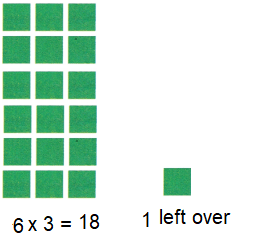Question 6.Quotient is 6 and remainder is 5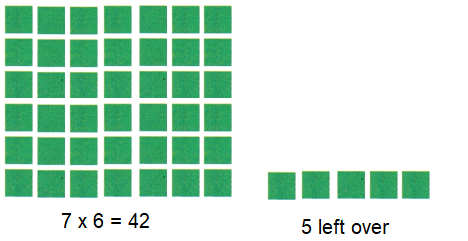Question 7.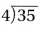Quotient is 8 and remainder is 3
Explanation: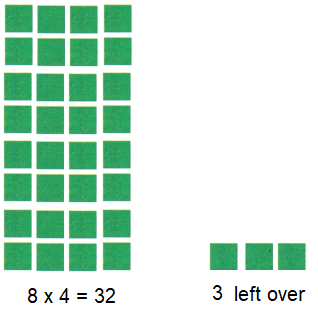Question 8.
23 ÷ 8
Quotient is 2 and remainder is 7
Explanation: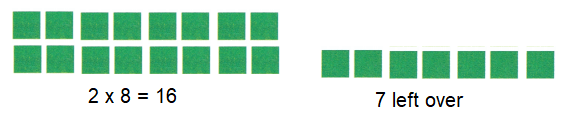Problem SolvingH.O.T. Multi-Step What’s the Error?Quotient and remainder are displayed together.
In this model only left over is shown separately.
Explanation:
13 ÷ 4
= 12 + 1
= 3 x 4 + 1
Question 9.
Macy, Kayley, Maddie, and Rachel collected 13 marbles. The want to share the marbles equally. How many marbles will each of the 4 girls get? How many marbles will be left over?
Quotient is 3 and remainder is 1
Explanation:Frank used an array to solve this problem. He says his array represents. What is his error?13 ÷ 4
Quotient is 3 and remainder is 1Explanation:
This model is easy to apply operation on different numbers of elements of an array.
First, we provide values like the number of elements and array.
Then a number
to divide all elements of an array using a for a loop.
Then we are going
to display the final numbers.
When a number cannot be divided evenly, the amount left over is called the remainder.

Question 10.
H.O.T. Explain how you use an array to find the quotient and remainder.
First, we provide values like the number of elements and array.
Then a number
to divide all elements of an array using a for a loop.
Then we are going
to display the final numbers.
When a number cannot be divided evenly, the amount left over is called the remainder.
Explanation:Question 11.
Jordyn explains to her friends how to play checkers. She has 29 minutes to explain to 3 teams. She wants to take the same number of whole minutes to explain to each team. What is the greatest number of whole minutes she can take to explain the rules to each team?
(A) 8 minutes
(B) 4 minutes
(C) 9 minutes
(D) 10 minutes
Option(C)
Explanation:
Jordyn has 29 minutes to explain to 3 teams.
She wants to take the same number of whole minutes to explain to each team.
29 ÷ 3 = 9 and 5 minutes is left over.
The greatest number of whole minutes she can take to explain the rules to each team is 9

Question 12.
Use Tools Kenny wants to put 37 books on 5 shelves. He wants the same number of books on each shelf and he will put the remaining books in a box. How many books does Kenny put in the box? Use tiles or draw an array to help you find the number of books Kenny put in the box.
(A) 6 books
(B) 2 books
(C) 7 books
(D) 3 books
Option(C)
Explanation:
Kenny wants to put 37 books on 5 shelves.
37 ÷ 5 = 7 ; 2 is left.
Number of books Kenny put in the box 7Question 13.
Multi-Step Barb puts 60 games into boxes to donate. Each box can hold 7 games. How many more games does Barb need so that all the boxes can have 7 games?
(A) 3 games
(B) 8 games
(C) 2 games
(D) 9 games
Option(A)
Explanation:
Barb puts 60 games into boxes to donate.
Each box can hold 7 games.
Total boxes 60 ÷ 7 = 8 is quotient and 4 is remainder.
Number of games Barb need so that all the boxes can have 7 games
7 – 4 = 3

TEXAS Test Prep

Question 14.
Rena has 23 DVDs that she wants to sort into rows of 6 each. How many DVDs will Rena have left over?
(A) 3
(B) 5
(C) 17
(D) 4
Option(B)
Explanation:
Rena has 23 DVDs that she wants to sort into rows of 6 each.
23 ÷ 6 = 3 quotient and 5 is remainder.
5 DVDs are left over with Rena.

### Texas Go Math Grade 4 Lesson 9.1 Homework and Practice Answer Key

Use tiles or draw an array to find the quotient and remainder.

Question 1.
47 ÷ 6 the quotient and remainder
47 ÷ 6 the quotient 7, and remainder 5
Explanation: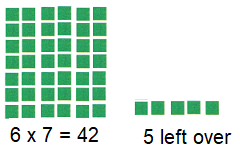Question 2.
38 ÷ 7
Quotient is 5
Remainder is 3
Explanation:38 ÷ 7 the quotient 5, and remainder 3

Question 3.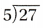Quotient is 5
Remainder is 2
Explanation: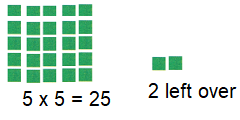Question 4.
30 ÷ 4
Quotient is 7
Remainder is 2
Explanation:Question 5.
23 ÷ 6
Quotient is 3
Remainder is 5
Explanation:Question 6.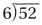Quotient is 8
Remainder is 4
Explanation: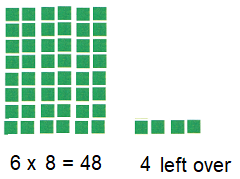Question 7.
34 ÷ 7
Quotient is 4
Remainder is 6
Explanation:Question 8.
18 ÷ 5
Quotient is 3
Remainder is 3
Explanation:Question 9.Quotient is 7
Remainder is 1
Explanation: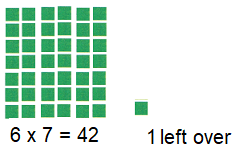Question 10.
23 ÷ 4
Quotient is 5
Remainder is 3
Explanation: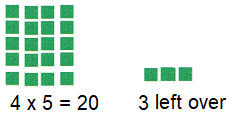Question 11.
35 ÷ 4
Quotient is 8
Remainder is 3
Explanation: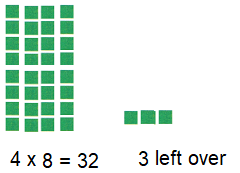Question 12.Quotient is 4
Remainder is 6
Explanation: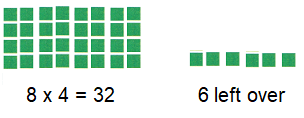Question 13.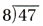Quotient is 5
Remainder is 7
Explanation: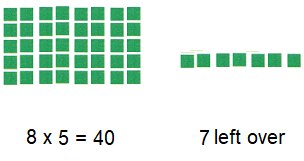Question 14.
27 ÷ 7
Quotient is 3
Remainder is 6
Explanation: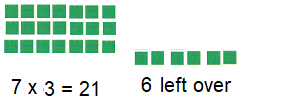Question 15.
19 ÷ 4
Quotient is 4
Remainder is 3
Explanation:Question 16.Quotient is 4
Remainder is 7
Explanation: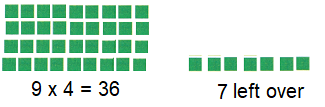Problem Solving

Question 17.
Miguel has 48 pieces of quartz. He wants to divide the pieces equally into 9 bags. How many pieces of quartz will be in each bag? How many pieces will be left over? Use tiles or draw an array to help you find the answer.

Each bag will have ___________ pieces and ___________ pieces will be left over.
Each bag will have 5 pieces and 3 pieces will be left over
Explanation:
Miguel has 48 pieces of quartz.
He wants to divide the pieces equally into 9 bags.
48 ÷ 9 = 5 is quotient and 3 is remainder.Question 18.
Lacy has 65 tickets for the school carnival. She and 6 friends will share the tickets equally. How many tickets will each person receive? How many tickets will be left over? Use tiles or draw an array to help you find the answer.

Each person will get ___________ tickets and ___________ tickets will be left over.
Each person will get 9 tickets and 2 tickets will be left over.
Explanation:
Lacy has 65 tickets for the school carnival.
She and 6 friends will share the tickets equally.
65 ÷ 7 = 9 is quotient and 2 is remainder.Lesson Check

Question 19.
Rebecca made 36 cookies for the school, bake sale. If she puts 5 cookies in a bag, how many bags will she use?
(A) 6
(B) 5
(C) 8
(D) 7
Option(D)
Explanation:
If she puts 5 cookies in a bag,
Total bags she use
36 ÷ 5 = 7

Question 20.
Felix has 60 towels to stack on a store shelf. He puts 8 towels in each stack. He puts any remaining towels in a drawer below the shelf. How many towels does Felix put in the drawer?
(A) 7
(B) 4
(C) 6
(D) 5
Option(A)
Explanation:
Felix has 60 towels to stack on a store shelf.
He puts 8 towels in each stack.
Total stacks she arranges 60 ÷ 8 = 7 is quotient and 3 is remainder
He puts 3 remaining towels in a drawer below the shelf.
7 towels Felix put in the drawer.

Question 21.
Lynn is packing 52 books for her family’s move. Each box can hold 9 books. What is the least number of boxes Lynn will need to pack all of the books?
(A) 7
(B) 8
(C) 6
(D) 5
Option(C)
Explanation:
Lynn is packing 52 books for her family’s move.
Each box can hold 9 books.
52 ÷ 9 = 5 is quotient and 7 is remainder
The least number of boxes Lynn will need to pack all of the books,
as 7 is left , he can pack 6 boxes.

Question 22.
Paul has 39 vocabulary cards to study. If he puts the cards in groups of 6, how many groups will he have, and how many cards will he have left over, if any?
(A) 6 groups with 3 cards left over
(B) 6 groups with 4 cards left over
(C) 6 groups with no cards left over
(D) 5 groups with 4 cards left over
Option(A)
Explanation:
Paul has 39 vocabulary cards to study.
If he puts the cards in groups of 6,
39 ÷ 6 = 6 is quotient and 3 is remainder
He have 6 groups and 3 cards left over.

Question 23.
Multi-Step Alyson has 46 beads to make bracelets. Each bracelet has 5 beads. How many more beads does Alyson need so that all the beads she has are used?
(A) 9
(B) 4
(C) 1
(D) 5
Option(B)
Explanation:
Alyson has 46 beads to make bracelets.
46 ÷ 5 = 9 is quotient and 1 is remainder.
4 more beads Alyson need so that all the beads she has are used,
as each bracelet has 5 beads.

Question 24.
Multi-Step Brad has saved $23 to spend on posters for his room. If each poster costs$4, how much more money does Brad need to save to buy 6 posters?
(A) $6 (B)$4
(C) $3 (D)$1
Brad has saved $23 to spend on posters for his room. If each poster costs$4,
So, 6 x 4 = $24 Total money Brad need to save to buy 6 posters 24 – 23 =$1